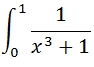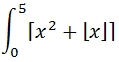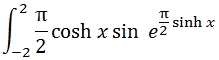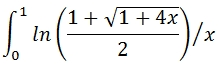# XLeratorDB/math Documentation

Updated: 31 March 2014

Use the scalar function QUADTS to evaluate a finite integral. QUADTS calculates the integral of the given function f(x) over the interval (a,b) using the Tanh-Sinh method.
Syntax
<@Func, nvarchar(max),>
,<@VarName, nvarchar(4000),>
,<@A, float,>
,<@B, float,>)
Arguments
@Func
the function to be integrated. @Func is a string containing any valid TSQL statement which includes a single variable that is the object of the integration. The variable name is defined in @VarName. @Func is of a type nvarchar or of any type which implicitly converts to nvarchar.
@VarName
the TSQL variable name. The variable name must start with '@'. @VarName must be of a type nvarchar or of a type which implicitly converts to nvarchar.
@A
The lower limit of integration. @A must be of a type float or of a type that implicitly converts to float.
@B
The upper limit of integration. @B must be of a type float or of a type that implicitly converts to float.
Return Types
float
Remarks
·         If @A not less than @B an error will be returned.
·         If @Func contains an undeclared SQL variable and it is not defined in @VarName a NULL will be returned.
Example
In this example we want to evaluate the integral:SELECT
'SELECT 1/(POWER(@x,3) + 1)', --@Func
'@x',                   --@VarName
0,                      --@A
1                       --@B
) as Integral

This produces the following result.
Integral
----------------------
0.835648848264721

In this example we want to evaluate the integral:SELECT
'SELECT CEILING(POWER(@x,2)+FLOOR(@x))', --@Func
'@x',                   --@VarName
0,                      --@A
5                       --@B
) as Integral

This produces the following result.
Integral
----------------------
54.3696455166722

In this example we want to evaluate the integral:Note that COSH, the hyperbolic cosine function, and SINH, the hyperbolic sine function, are not a built-in SQL Server functions but are part of the XLeratorDB library.
SELECT
'SELECT PI()/2e+00*wct.COSH(@x)*SIN(EXP(PI()/2e+00*wct.SINH(@x)))',                 --@Func
'@x',                   --@VarName
-2,                     --@A
2                       --@B
) as Integral

This produces the following result.
Integral
----------------------
1.57044006384986

In this example we want to evaluate the integral:SELECT
'SELECT LOG((1+SQRT(1+4*@x))/2)/@x',      --@Func
'@x',             --@VarName
0,                --@A
1                 --@B
) as Integral

This produces the following result.
Integral
----------------------
0.657973626739288

### SupportCopyright 2008-2023 Westclintech LLC         Privacy Policy        Terms of Service